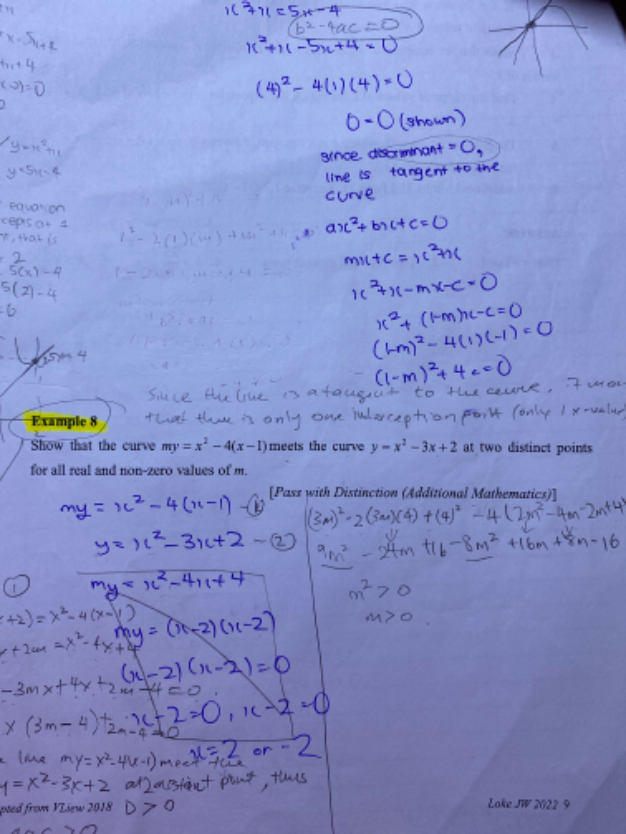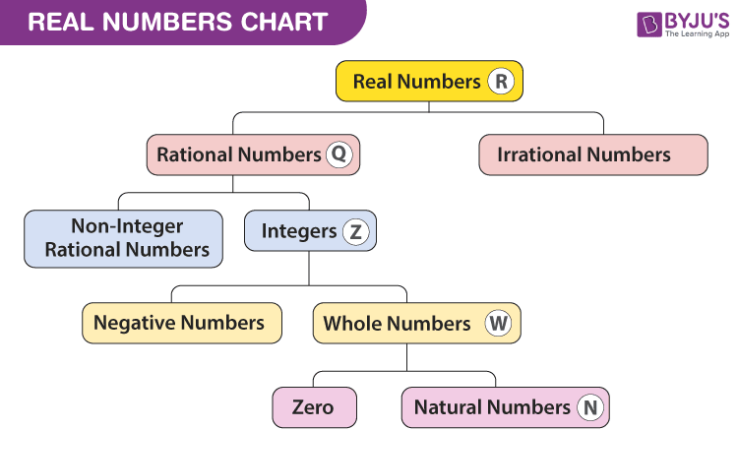# QuestionKindly help me with example 8 thanksFirstly, you know that:

b2 – 4ac > 0

If the discriminant is greater than zero, this means that the quadratic equation has two real, distinct (different) roots.

m(x2 – 3x + 2 ) = x2 -4x + 4

mx -3mx + 2m =  x2 -4x + 4

(m-1)x2  -(3m-4)x + (2m-4) = 0

b2 – 4ac > 0     for 2 real and distinct roots

=>   [-(3m-4)] – 4(m-1)(2m-4)  > 0

9m2 -24m + 16 – 4(2m2 – 6m + 4)  > 0

9m2 -24m + 16 – 8m2 + 24m – 16 > 0

m2 > 0    =>    m is non-zero and real numbers(includes rational and irrational, positive and negative, see the above chart for a comprehensive definition of real numbers)